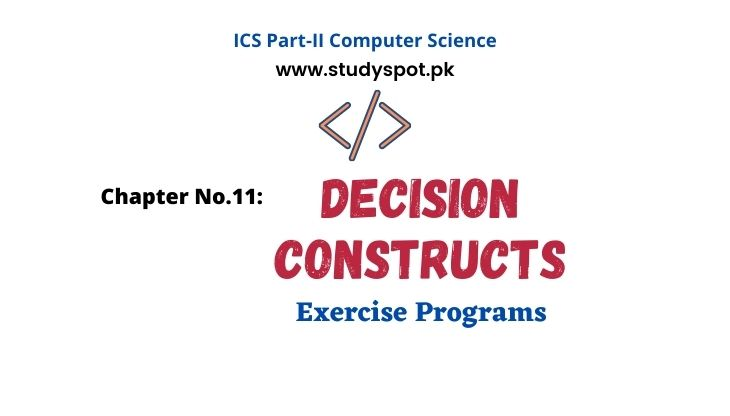# Decision Making Statements in CDecision Making Statements in CThis post for Computer ICS Part 2 | Computer Science 2nd Year Chapter No.11 Decision Constructs. Find the solution to all exercise questions in C language.  Following exercise programs in C are important for the final exams.

We tried our best to provide the best solution of exercise programs of Chapter No.11: Decision Constructs in C language. This post covers the topics i.e. control structures (sequence, selection, and loop), simple if statement, if-else statement, nested if statement, if-else if statement, comparison of nested if & sequence of ifs statements, switch statement, and conditional operator.

### Chapter No.11: Decision Constructs

#### Exercise Programs

Q.8: Write an interactive program that contains an if statement that may be used to compute the area of a square (area=side^2) or a triangle (area=1/2\times base \times height) after prompting the user to type the first character of the figure name (S or T).

#include<stdio.h>

#include<conio.h>

void main(void)

{

char ch;

float area,side,base,height;

printf("Enter S:area of square T:area of triangle:");

ch=getche();

if(ch=='S' || ch=='s')

{

printf("\nEnter the value of side of square:");

scanf("%f",&side);

area=side*side;

printf("\nArea of Square:%.2f", area);

}

else if(ch=='T' || ch=='t')

{

printf("\nEnter the value of base of triangle:");

scanf("%f",&base);

printf("\nEnter the value of height of triangle:");

scanf("%f",&height);

area=(base*height)/2.0;

printf("\nArea of Triangle:%.2f", area);

}

else

printf("\nWrong choice...\n\t\t Please enter S or T");

}

Q.9: A year is a leap year if it is divisible four, except that any year divisible by 100 is a leap only if it is divisible by 400. Write a program that inputs a year such as 1996, 1800, and 2010, and displays “Leap year” if it is a leap year otherwise displays “Not a leap year”.

#include<stdio.h>

#include<conio.h>

void main(void)

{

int year;

printf("\tEnter Year=");

scanf("%d",&year);

if(year%4==0)

printf("\n\t%d is a Leap Year! ",year);

else

printf("\n\t%d is not a Leap Year!",year);

}

Q.10: Write a program that inputs temperature and displays a message according to the following data:

 Temperature Message Greater than 35 Hot day Between 25 and 35 (inclusive) Pleasant day Less than 25 Cool day

#include<stdio.h>

#include<conio.h>

void main(void)

{

int temp;

printf("\t\tEnter temperature=");

scanf("%d",&temp);

if(temp>35)

printf("\n\tHot day! ");

else if(temp>=25 && temp<=35)

printf("\n\tPleasant day!");

else

printf("\n\tCool day !");

}

Q.11: Write a program that inputs obtained marks of a student, calculate the percentage (assume total marks are 1100), and displays his/her grade. The grade should be calculated according to the following rules:

 Percentage Grade More than or equal to 80 A+ Between 70 (inclusive) and 80 A Between 60 (inclusive) and 70 B Between 50 (inclusive) and 60 C Between 40 (inclusive) and 50 D Between 33 (inclusive) and 40 E Less than 33 F

#include<stdio.h>

#include<conio.h>

void main(void)

{

int obt_marks;

float page;

printf("Enter Obtained Marks = ");

scanf("%d",&obt_marks);

page=(obt_marks*100)/1100;

printf("\n\tPercentage : %.0f",page);

if(page>=80)

else if(page>=70 && page<80)

else if(page>=60 && page<70)

else if(page>=50 && page<60)

else if(page>=40 && page<50)

else if(page>=33 && page<40)

else

printf("\n\tF");

}

Q.12: Write a program that inputs two numbers and asks for the choice of the user, if user enters 1 then displays the sum of numbers, if user enters 2 then displays result of subtraction of the numbers. If user enters 3 then displays the result of the multiplication of the numbers and if user enters 4 then displays result of the division of the numbers (divide the larger number by the smaller number), otherwise displays the message “Wrong Choice”. [Use a switch statement to implement the solution]

#include<stdio.h>

#include<conio.h>

void main(void)

{

int choice;

float a,b;

printf("Enter First No.?");

scanf("%f",&a);

printf("\nEnter Second No.?");

scanf("%f",&b);

scanf("%d",&choice);

switch(choice)

{

case 1:

break;

case 2:

printf("\n\tSubtraction Result=%.2f\n\n",a-b);

break;

case 3:

printf("\n\tMultiplication Result=%.2f\n\n",a*b);

break;

case 4:

if(a>b)

printf("\n\tDivision Result=%.2f\n\n",a/b);

else

printf("\n\tDivision Result=%.2f\n\n",b/a);

break;

default:

printf("\n\tWrong choice...");

}

}

### The following may relate to you!

➤ Decision Constructs - MCQs

➤ Decision Constructs - Short Questions